# Live RUL Estimation of a Servo Gear Train using ThingSpeak

This example shows how to estimate the Remaining Useful Life (RUL) of a servo motor gear train through real-time streaming of servo motor data from an Arduino-based data acquisition system to ThingSpeak and from ThingSpeak to an RUL estimation engine running in MATLAB.

Motor current signature analysis (MCSA) of the current signal driving a hobby servo motor is used to extract frequency-domain (spectral) features from several frequency regions of interest indicative of motor and gear train faults. A combination of features are used construct a Health Indicator (HI) for subsequent RUL estimation.

MCSA is a useful method for the diagnosis of faults that induce torque or speed fluctuations in the servo gear train, which in turn result in correlated motor current changes. MCSA has been proven to be ideal for motor fault analysis as only the motor current signal is required for analysis, which eliminates the need for additional and expensive sensing hardware. Gear fault detection using traditional vibration sensors is challenging, especially in cases where the gear train is not easily accessible for instrumentation with accelerometers or other vibration sensors.

This example illustrates how to build a real-time data streaming, feature extraction, and RUL estimation system using simple, off-the-shelf components suitable for educational lab exercises or for prototyping of industrial applications. To run this example without hardware, use the `sendSyntheticFeaturesToThingSpeak` function to generate synthetic data.

The simplified workflow to construct the data streaming system and the RUL estimation engine includes the following steps:

1. Develop the hardware and the data acquisition system using off-the-shelf components.

2. Stream real-time data from a microcontroller like an Arduino UNO to MATLAB.

3. Process the real-time data in MATLAB to extract features and stream the features to the cloud using ThingSpeak.

4. Stream the cloud data to an RUL estimation and visualization engine in MATLAB.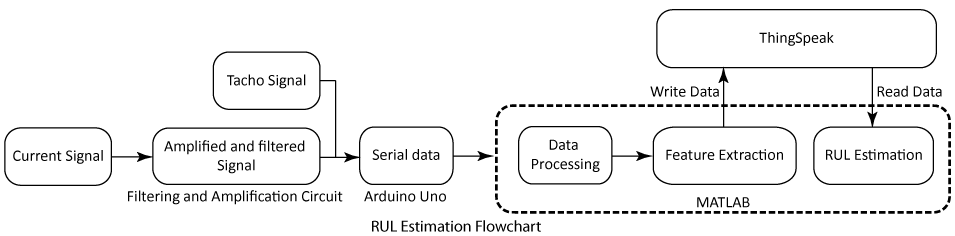### Hardware Setup and Data Acquisition

#### Hardware Overview

For this example, the electrical current data was collected from a standard Futaba S3003 hobby servo, which was modified for continuous rotation. Servos convert the high speed of an internal DC motor to high torque at their output shaft. To achieve this, servos consist of a DC motor, a set of nylon or metal gear pairs, and a control circuit. The control circuit was removed to be able to apply a constant 5V voltage to the DC motor leads directly and allow the current through the DC motor to be monitored through a current sensing resistor.A second, opposing servo with its DC motor terminals shunted together through a low-value resistor was used to generate a high-torque opposing load for the driver servo to speed up the degradation of the gear train and induce larger variations in the motor current signal for MCSA analysis.

#### Servo Motor and Gear Train

The Futaba S3003 servo consists of four pairs of meshing nylon gears as illustrated in the figure below. The pinion P1 on the DC motor shaft meshes with the stepped gear G1. The pinion P2 is a molded part of the stepped gear G1 and meshes with the stepped gear G2. The pinion P3, which is a molded part of gear G2, meshes with the stepped gear G3. Pinion P4, which is molded with G3, meshes with the final gear G4 that is attached to the output shaft. The stepped gear sets G1 and P2, G2 and P3, and G3 and P4 are free spinning gears $-$ that is, they are not fixed to their respective shafts. The set of gears provides a 278:1 reduction going from a motor speed of about 6117 rpm to about 22 rpm at the output shaft when the DC motor is driven at 5 volts.The following table outlines the tooth count and theoretical values of output speed, gear mesh frequencies, and cumulative gear reduction at each gear mesh.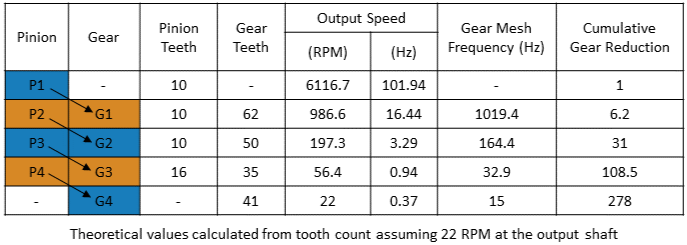The rotation speed of the output shaft of the servo was measured using an infrared photointerrupter along with a 40 mm diameter, 3-D printed tacho wheel with 16 slots. The sixteen slots on the wheel are equally spaced and the IR photointerrupter was placed such that there were exactly sixteen pulses per rotation of the wheel. The servos and the photointerrupter were held in place by 3-D printed mounts.The driving DC motor was driven at a constant 5 volts, and with four pairs of gears providing 278:1 speed reduction, the output shaft speed had an average value of 22 rpm. The fluctuations of output speed and the corresponding changes in the motor current due to gear meshing and gear teeth degradation over time were captured by sensors for further analysis in MATLAB.

#### Filtering and Amplification Circuit

The current consumption through the DC motor windings was calculated using Ohm's law by measuring the voltage drop across a 0.5-ohm, 0.5 W resistor. Since the change in current measurement values was too small for high-quality detection, the current signal was amplified 20 times using a single-supply sensor interface amplifier (Analog Devices AD22050N). The amplified current signal was then filtered using an anti-aliasing fifth-order elliptic low-pass filter (Maxim MAX7408) to smooth it and to eliminate noise before sending it to an analog-to-digital converter (ADC) pin on the Arduino UNO. The corner frequency of the anti-aliasing filter was placed at around 470 Hz to provide ample attenuation at the Nyquist frequency (750 Hz) of the analog-to-digital converter.

The electronic circuit was realized on a prototype printed circuit board (PCB) using a small number of additional passive components as shown in the picture below.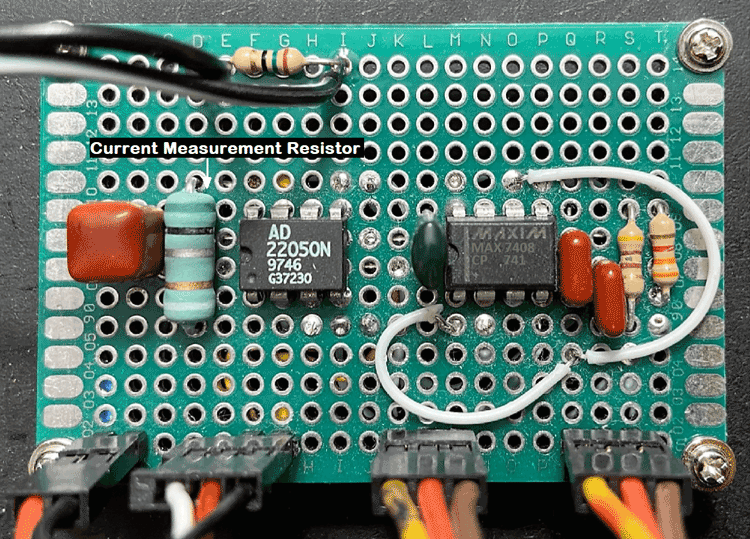As the flowchart shows below, Arduino UNO sampled the current signal through an ADC input at a 1500 Hz sampling frequency and streamed it to the computer, along with the tachometer pulses, over a serial communication channel running at a baud rate of 250000 bps.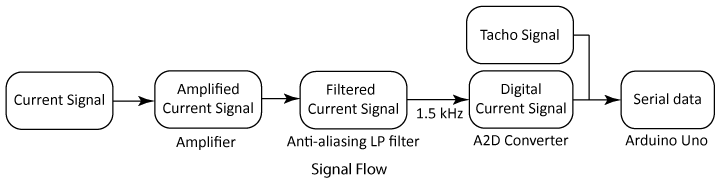For the data in this example, the serial signal was encoded on the Arduino UNO as three 8-bit binary values per data sample (current + tacho) to improve the serial communication efficiency with the computer.

### Real-time Data Streaming

#### Streaming Data from Arduino UNO to MATLAB

The Arduino UNO encoded the motor current and the tacho pulse values into a binary buffer for efficiency and sent batches of data to the computer consisting of 16384 (2^14) samples every 5 minutes. The data was sent from the Arduino UNO at a rate of 1500 Hz using a simple data protocol consisting of start and end markers around the data bytes for easy extraction of data batches in MATLAB code.

See the attached Arduino sketch file `servo_data_producer.ino` for more information and to stream data to MATLAB from your hardware setup.

#### Receiving Live Data Stream in MATLAB

You can use a MATLAB script to fetch batches of serial data from the Arduino UNO with the appropriate baud rates and serial COM port numbers.

First, open the serial port for communication with your Arduino or equivalent hardware. You can use the `serialportlist` command to find the serial COM port. For this example, the Arduino UNO is on `COM4`. Use a baud rate of 250000 bps.

```port = "COM4"; baudRate = 250000; ```

Set an appropriate timeout and use the correct data terminator from the Arduino code contained in the file `servo_data_producer.ino`. For this example, use a timeout of 6 minutes. Then, clear the serial port buffer using `flush`.

```s = serialport(port,baudRate,'Timeout',360); configureTerminator(s,"CR/LF"); flush(s); ```

Now, read and process the encoded data stream from the Arduino with an appropriate number of read cycles. For this example, use 10 read cycles. For more details, see the supporting function `readServoDataFromArduino` provided in the Supporting Functions section.

```count = 0; countMax = 10; while (count < countMax) try count = count + 1; fprintf("\nWaiting for dataset #%d...\n", count); readServoDataFromArduino(s); catch e % Report any errors. clear s; throw(e); end end ```

Now, use the `clear` command to close the serial port.

```clear s; ```

### Data Processing and Feature Extraction in MATLAB

#### Processing Live Stream Data

Once the data is streamed into MATLAB, you can use the `readServoDataFromArduino` script to construct a data matrix to store the latest batch of servo motor data. For more details, see the supporting function `readServoDataFromArduino` provided in the Supporting Functions section.

```readServoDataFromArduino(s) ```

The resultant timetable contains the time stamp, motor current, and tacho pulse values for the latest dataset streamed from the Arduino UNO.

Use the `processServoData` function to output a timetable with relevant physical units.

```T = processServoData(X) ```

The resulting timetable provides a convenient container for extracting predictive features from the motor data.

If you do not have the necessary hardware, you can run this example using the `sendSyntheticFeaturesToThingSpeak` function to generate synthetic data.

#### Feature Extraction

From each new dataset, compute the nominal speed to detect frequencies of interest in the gear train and match them correctly with the frequencies on their power spectra.

```F = extractFeaturesFromData(T); ```

Using the sampling frequency value of 1500 Hz in the `tachorpm` command, compute the nominal speed of the output shaft. For this instance, the RPM value is constant around 22 rpm.

```tP = T.TachoPulse; rpm = tachorpm(tP,Fs,'PulsesPerRev',16,'FitType','linear'); rpm = mean(rpm); ```

The motor speed along with the physical parameters of the gear sets, enable the construction of fault frequency bands, which is an important prerequisite for computing the spectral metrics. Using the tooth count of the drive gears in the gear train and the nominal rpm, first compute the frequencies of interest. The frequencies of interest are the actual output speed values in Hertz whose values were close to the theoretical values listed in the table below.Next, construct the frequency bands for the all the output speeds which include the following frequencies of interest using the `faultBands` command. The selected fundamental frequencies, harmonics, and sidebands are discussed in more details in the Analyze Gear Train Data and Extract Spectral Features Using Live Editor Tasks example. In particular, the important fault frequencies to monitor in the servo system were identified as follows:

• First six harmonics of FS1 with 0:1 sidebands of FS2

• 1st, 2nd, and 4th harmonics of FS2 with 0:1 sidebands of FS3

• 3rd harmonic of FS3

```% Number of gear and pinion teeth. G4 = 41; G3 = 35; G2 = 50; G1 = 62; P4 = 16; P3 = 10; P2 = 10; P1 = 10; % Shaft speeds in Hz. FS5 = rpm / 60; FS4 = G4 / P4 * FS5; FS3 = G3 / P3 * FS4; FS2 = G2 / P2 * FS3; FS1 = G1 / P1 * FS2; % Generate the fault bands of interest. FB_S1 = faultBands(FS1, 1:6, FS2, 0:1); FB_S2 = faultBands(FS2, [1 2 4], FS3, 0:1); FB_S3 = faultBands(FS3, 3); FB = [FB_S1; FB_S2; FB_S3]; ```

Use the `faultBandMetrics` command along with the power spectral density (`pwelch` command) of the motor current signal to compute a total of 85 spectral metrics.

Compute the power spectrum of the motor current data.

```mC = T.MotorCurrent; [ps,f] = pwelch(mC,[],[],length(mC),Fs); ```

Compute spectral metrics for all fault bands.

```metrics = faultBandMetrics(ps,f,FB); metrics = metrics{:, [4 13 85]}; % Select significant metrics. ```

Pick features to track out of the metrics computed. Select some of the significant ones such as the peak amplitude of the spectrum at the first and second harmonics of the shaft 1 speed (FS1).

```features = [metrics,mean(mC),rpm]; ```

Note that the average motor current and the average speed in rpm of the motor are also recorded as additional features.

### Live Data Streaming and RUL Estimation

#### Store Feature Data in Cloud

Once various features are computed from real-time data in batches every 5 minutes, send them to ThingSpeak as part of the same MATLAB script for cloud storage and remaining useful life analysis.

Send feature values to a ThingSpeak channel as separate fields using the `sendFeaturesToThingSpeak` function.

```sendFeaturesToThingSpeak(features) ```

Here is an example of a ThingSpeak channel that is configured with a number of fields to store the feature values. The channel serves as a convenient data repository and visualization platform.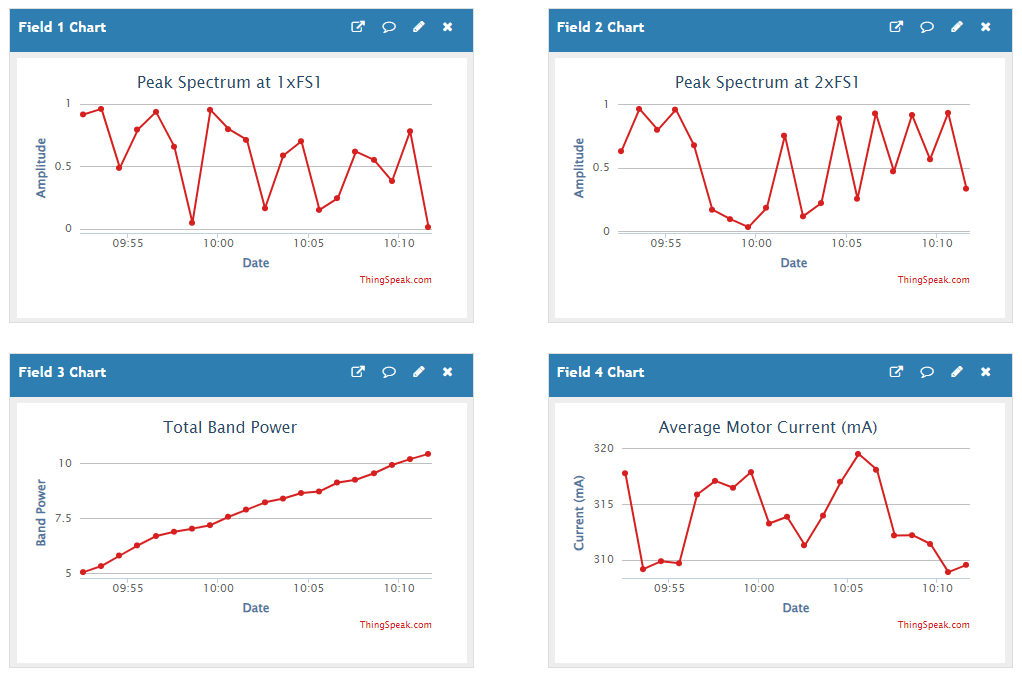The ThingSpeak channel provides a real-time feature monitoring dashboard for tracking the changes in feature values. It also acts as the data source for feature processing such as remaining useful life estimations.

If you don't have the hardware setup to generate features from the servo motor, you can generate synthetic feature values to illustrate the computation of subsequent RUL estimates using the following code example. You can have separate instances of MATLAB running on different machines to read and write data to ThingSpeak.

```hasArduino = false; % Set to true when using Arduino UNO. if ~hasArduino % Set timer to send new feature data every minute. tmr1 = timer('ExecutionMode','fixedSpacing','Period',60); tmr1.TimerFcn = @(~,~) sendSyntheticFeaturesToThingSpeak(); tmr1.start(); return; end```

#### Read Feature Data from Cloud

A separate MATLAB script, which can also be deployed as a compiled MATLAB app or as a deployed Web App, can be used to read the servo motor features from ThingSpeak and compute real-time estimates of the RUL metric. For this instance, construct an initial exponential degradation model and update the model periodically as new feature values become available.

```% Set timer to check for new data every minute and update the RUL model, mdl. tmr2 = timer('ExecutionMode','fixedSpacing','Period',60); tmr2.TimerFcn = @(~,~) readFeaturesFromThingSpeak(mdl,channelID,readKey); tmr2.start(); ```

Each new set of features are used to update the exponential degradation model and compute real-time estimates of the RUL.

#### Live Remaining Useful Life Estimation

For this example, assume that the training data is not historical data, but rather real-time observations of the component condition as captured by the features computed in the previous steps. An exponential degradation model (`exponentialDegradationModel`) is suitable for this type of real-time RUL estimation and visualization. The RUL model uses a health index to indicate the system of the system and a threshold to estimate the RUL of the system. For this example, the threshold is on the `Total Band Power` feature of the servo motor as computed in the previous steps. The total band power captures the changes in the spectral energy within the fault band frequencies associated with important frequency regions of the servo motor gear train.

Construct the exponential degradation model with an arbitrary prior distribution data and a specified noise variance.

Specify the lifetime and data variable names for the observation data from a subset of the data table read from ThingSpeak. Then, use each feature values to predict the RUL of the component using the current life time value stored in the model.

```channelID = 1313749; % Replace with the channel ID to write data to. readKey = 'KYIDUZ1ENDT3TGG0'; % Replace with the read API key of your channel. % Read most recent degradation data from ThingSpeak. T = thingSpeakRead(channelID,'ReadKey',readKey,'OutputFormat','timetable'); % Construct the exponential degradation model using Total Band Power as the % health index. mdl = exponentialDegradationModel( ... 'Theta', 1, 'ThetaVariance', 100, ... 'Beta', 1, 'BetaVariance', 100, ... 'NoiseVariance', 0.01, ... 'LifeTimeVariable', T.Properties.DimensionNames{1}, ... 'DataVariables', T.Properties.VariableNames{3}, ... 'LifeTimeUnit', "hours"); % Set timer to check for new data every minute. tmr2 = timer('ExecutionMode','fixedSpacing','Period',60); tmr2.TimerFcn = @(~,~) readFeaturesFromThingSpeak(mdl,channelID,readKey); tmr2.start();```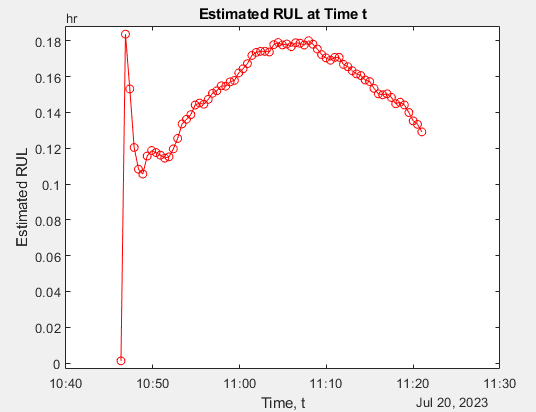The MATLAB script tracks the feature data available on the ThingSpeak channel and updates the RUL model as new feature values become available, thereby providing a real-time RUL estimation and visualization capability.

### Supporting Functions

The supporting function `readServoDataFromArduino` reads and processes the encoded data streams from the Arduino UNO.

```function readServoDataFromArduino(s) % Reads and processes encoded data streams from Arduino. % Find start of encoded data stream. str = readline(s); N = sscanf(str, "BEGIN:%d"); % Expected number of data points (should be 16384). % Acquire new data until the end of data stream. if ~isempty(N) && N > 0 X = zeros(N,2); % Read and store encoded binary data. disp("Reading servo motor data..."); for k = 1:N % Read three bytes each time containing motor current and tacho pulse values. x = [read(s,1,"uint16"), read(s,1,"uint8")]; X(k,:) = double(x); end % Find end of encoded data stream. readline(s); % Remove CR/LF from data stream. str = readline(s); if str == "END" disp("Processing servo data..."); T = processServoData(X); disp('Extracting features...'); F = extractFeaturesFromData(T); disp('Sending features to ThingSpeak...'); sendFeaturesToThingSpeak(F); else error('Error reading end-of-file marker from serial port.'); end end end```

The supporting function `processServoData` converts raw data matrices to a timetable with appropriate variable names and physical units.

```function T = processServoData(X) % Converts raw data matrix to a timetable with appropriate variable names and % physical units. Fs = 1500; % Sampling rate. Rsens = 0.5; % Current sense resistor value in ohm. Kamp = 20; % Sensor amplifier gain. count2volt = 5/1024; % Scaling factor between digital reading to analog voltage. T = timetable('SampleRate', Fs); T.MotorCurrent = X(:,1) * count2volt / Kamp / Rsens * 1000; % Convert to mA. T.TachoPulse = X(:,2); % On/off tacho pulse values. T.Properties.VariableUnits = {'mA', 'level'}; end```

The supporting function `extractFeaturesFromData` extracts features from time-domain servo motor data in table format.

```function features = extractFeaturesFromData(T) % Extracts up to 8 features from time-domain servo data provided in table % format. Fs = 1500; % Sampling rate. % Average motor speed. tP = T.TachoPulse; rpm = tachorpm(tP, Fs, 'PulsesPerRev', 16, 'FitType', 'linear'); rpm = mean(rpm); % Number of gear and pinion teeth. G4 = 41; G3 = 35; G2 = 50; G1 = 62; P4 = 16; P3 = 10; P2 = 10; P1 = 10; % Shaft speeds in Hz. FS5 = rpm / 60; FS4 = G4 / P4 * FS5; FS3 = G3 / P3 * FS4; FS2 = G2 / P2 * FS3; FS1 = G1 / P1 * FS2; % Generate the fault bands of interest. FB_S1 = faultBands(FS1, 1:6, FS2, 0:1); FB_S2 = faultBands(FS2, [1 2 4], FS3, 0:1); FB_S3 = faultBands(FS3, 3); FB = [FB_S1; FB_S2; FB_S3]; % Compute the power spectrum of the motor current data. mC = T.MotorCurrent; [ps,f] = pwelch(mC, [], [], length(mC), Fs); % Compute spectral metrics for all fault bands. metrics = faultBandMetrics(ps, f, FB); metrics = metrics{:, [4 13 85]}; % Select significant metrics. % Pick features to track. features = [metrics, mean(mC), rpm]; end```

The supporting function `sendFeaturesToThingSpeak` sends feature values to a ThingSpeak channel as separate fields.

```function sendFeaturesToThingSpeak(features) % Sends feature values to a ThingSpeak channel as separate fields. % Configure the Channel ID and the Write API Key values. channelID = 1313749; % Replace with your channel ID to write data to. writeKey = 'X96MRW1TTZC1XNV3'; % Replace with the write API key of your channel. % Write the features to the channel specified by the 'channelID' variable. fields = 1:numel(features); % Up to 8 features thingSpeakWrite(channelID, features, 'Fields', fields, 'WriteKey', writeKey); end```

The supporting function `readFeaturesFromThingSpeak` reads feature values from a ThingSpeak channel as new data becomes available by tracking the time stamps of the latest data readings. The function also updates the RUL model using the new feature values and plots the predicted RUL values.

```function readFeaturesFromThingSpeak(mdl, channelID, readKey) % Read feature values from ThingSpeak to update the RUL estimation. persistent timestamp persistent hplot if isempty(timestamp) % Start with last ten days of data. timestamp = dateshift(datetime, "start", "day", -10); end % Read recent data from all fields. disp('Reading features from ThingSpeak...'); T = thingSpeakRead(channelID, 'ReadKey', readKey, ... 'OutputFormat', 'timetable', 'DateRange', [timestamp, datetime]); if ~isempty(T) T = T(T.Timestamps > timestamp, :); % Only keep most recent data. end if ~isempty(T) T = T(T.Timestamps > timestamp, :); % Only keep most recent data. timestamp = T.Timestamps(end); % Update time stamp for most recent data. % Set RUL degradation threshold. threshold = 10; % The training data is not historical data, but rather real-time observations % of the servo motor features. N = height(T); estRUL = hours(zeros(1,N)); for i = 1:N update(mdl, T(i,:)) estRUL(i) = predictRUL(mdl, threshold); end % Visualize RUL over time. if isempty(hplot) || ~isvalid(hplot) hplot = plot(T.Timestamps(:)', estRUL, 'r-o'); title('Estimated RUL at Time t') xlabel('Time, t') ylabel('Estimated RUL') else hplot.XData = [hplot.XData, T.Timestamps(:)']; hplot.YData = [hplot.YData, estRUL]; end end end```

When a servo motor and an Arduino UNO are not available to generate feature values, use the supporting function `sendSyntheticFeaturesToThingSpeak` to provide synthetic feature data to illustrate streaming of features values to ThingSpeak.

```function sendSyntheticFeaturesToThingSpeak() % Generate synthetic features and send them to ThinkSpeak. persistent bandPower if isempty(bandPower) bandPower = 5; end % Simulate motor features and fault band metrics. motorCurrent = 308+12*rand(1); % between [308 320] mA rpm = 20+3*rand(1); % between [20 23] rpm bandPower = bandPower + rand(1)/2; % bandPower degradation metrics = [rand(1), rand(1), bandPower]; features = [metrics, motorCurrent, rpm]; disp('Sending features to ThingSpeak...'); sendFeaturesToThingSpeak(features) end```